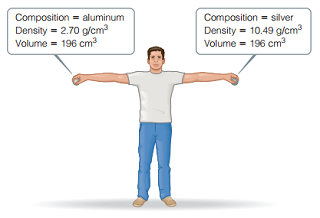# Problem: Consider the two spheres shown here, one made of silver and the other of aluminum.What is the mass of the aluminum sphere in kg?

###### FREE Expert Solution

$\overline{)\mathbf{density}\mathbf{=}\frac{\mathbf{mass}}{\mathbf{volume}}}$

density = 2.70 cm3

volume = 196 cm3

1 kg = 103 g

79% (301 ratings)###### Problem Details

Consider the two spheres shown here, one made of silver and the other of aluminum.What is the mass of the aluminum sphere in kg?

Frequently Asked Questions

What scientific concept do you need to know in order to solve this problem?

Our tutors have indicated that to solve this problem you will need to apply the Density concept. You can view video lessons to learn Density. Or if you need more Density practice, you can also practice Density practice problems.

What professor is this problem relevant for?

Based on our data, we think this problem is relevant for Professor Hidalgo's class at ARIZONA.